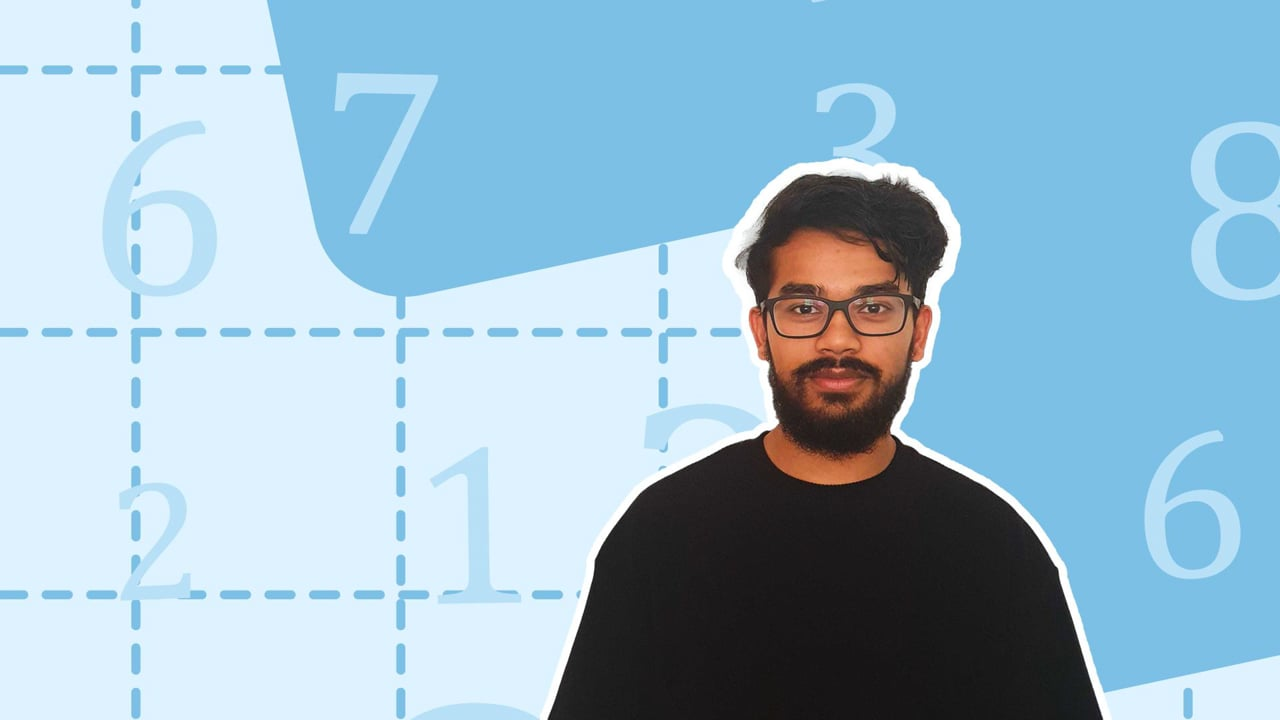Chapter overviewMaths

Number and place value

Multiplication and division

Fractions

Measurement

Geometry - properties of shapes

Geometry - position and direction

Statistics

Maths

# Square and cube numbers0%

Summary

# Square and cube numbers

## ​​In a nutshell

Square and cube numbers are given the name square and cube because the area of a square is a number multiplied by itself once whilst the volume of a cube is a number multiplied by itself twice. There are $10$​ square numbers and $4$​ cube numbers between $1$ and $100$.​

## Square numbers

Square numbers are made when a number is multiplied by itself once. The symbol to show a square number is $^2$ so, $3^2$ means $3$ squared or $3\times3$.​

##### Example 1

What are the first $5$ square numbers?

\begin{aligned}1^2 &= 1 \times 1 = 1 \\ 2^2 &= 2 \times 2 = 4 \\ 3^2 &= 3 \times 3 = 9 \\ 4^2 &= 4 \times 4 = 16 \\ 5^2 &= 5 \times 5 = 25 \end{aligned}

The first five square numbers are $\underline{1,4,9,16,25}$.

## Cube numbers

Cube numbers are made when a number is multiplied by itself twice . The symbol to show a cube number is $^3$ so, $3^3$ means $3$ cubed or $3\times3 \times 3$.

##### Example 2

What are the first $3$ cube numbers?

\begin{aligned}1^3 &= 1 \times 1 \times 1 = 1 \\ 2^3 &= 2 \times 2 \times 2= 8 \\ 3^3 &= 3 \times 3 \times 3 = 27 \end{aligned}​​

The first three cube numbers are $\underline{1,8,27}$.

FAQs

• Question: What is a cube number?

Answer: Cube numbers are made when a number is multiplied by itself twice .

• Question: What is a square number?

Answer: Square numbers are made when a number is multiplied by itself once.

Theory

Exercises